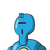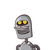# the length of rectamgular filled exceeds its breathe 9 meters if the perimeter of the Field is 154 m find lenght, breadth?​

the length of rectamgular filled exceeds its breathe 9 meters if the perimeter of the Field is 154 m find lenght, breadth?​

### 2 thoughts on “the length of rectamgular filled exceeds its breathe 9 meters if the perimeter of the Field is 154 m find lenght, breadth?​”

1.Perimeter = 2(1 + b) = 154m

Length = b + 9 (according to question)

1542((b + 9) + b)

154 = 2(2b + 9)

154/ 22b+9

77= 2b + 9

77-9=2b

68=2b

b = 68/2

b = 34m

1 = b + 9 = 34 + 9 = 43m

Step-by-step explanation:

Hi ARMY, I think you’re from Tamil Nadu can I be your friend? I am Niharika and I am also from Tamil Nadu 🙂

2.Breadth = 34m    Length = 43m

Step-by-step explanation:

Perimeter = 2(l + b) = 154m

Length  = b + 9  (according to question)

154 = 2((b + 9) + b)

154 = 2(2b + 9)

154/2 = 2b + 9

77 = 2b + 9

77 – 9 = 2b

68 = 2b

b = 68/2

b = 34m

l = b + 9 = 34 + 9 = 43m